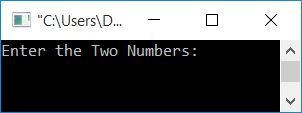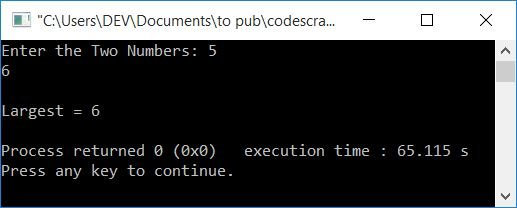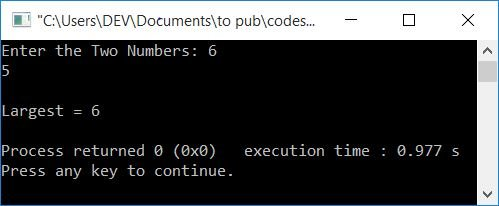# C++ Program to Find the Largest of Two Numbers

In this article, you will learn and get code to find and print the largest of two numbers given by the user at run-time in C++. Here is the list of approaches used to create the program:

## In C++, use if-else to find the larger of two numbers

To find the largest between two numbers in C++ programming, you have to ask the user to enter any two numbers. Now use the if-else statement to find the largest. and then print the largest as shown in the program given below.

The question is, "Write a program in C++ to find the largest or greatest of two numbers." Here is its answer:

```#include<iostream>
using namespace std;
int main()
{
int numOne, numTwo, larg;
cout<<"Enter the Two Numbers: ";
cin>>numOne>>numTwo;
if(numOne>numTwo)
larg = numOne;
else
larg = numTwo;
cout<<"\nLargest = "<<larg;
cout<<endl;
return 0;
}```

This program was built and runs under the Code::Blocks IDE. Here is its sample run:Now supply any two numbers, say 5 and 6. Press the ENTER key to find and print the largest one, as shown in the snapshot given below:Here's another sample run with user input, this time with 6 (as the first number) and 5 (as the second number):In the above program, using if-else, we've compared the value of numOne (the first number) with numTwo (the second number). That is, if numOne's value is greater than numTwo's, then the value of numOne gets initialized to larg. Otherwise, the value of numTwo is set to larg.

When the user enters the two numbers, say 5 and 6, in the same order, logic dictates that 5 as the first number and 6 as the second. Then 5 gets stored in numOne, and 6 gets stored in numTwo.

The condition of the if gets evaluated. That is, the condition numOne>numTwo or 5>6 evaluates to be false, therefore the program flow does not go inside the if's body. It instead goes to the else's body. And the value of numTwo (6) gets initialized to larg.

Finally, just print the value of larg on the output, that will be the largest number on your screen.

## In C++, use the conditional operator to find the greater of two numbers

Another C++ program that finds and prints the largest number between two given numbers, but this time using the conditional operator (?:).

```#include<iostream>
using namespace std;
int main()
{
int numOne, numTwo, larg;
cout<<"Enter the Two Numbers: ";
cin>>numOne>>numTwo;
larg = (numOne>numTwo) ? numOne : numTwo;
cout<<"\nLargest = "<<larg;
cout<<endl;
return 0;
}```

This program produces the same output as the previous program. In this program, the following code is used:

`(numOne>numTwo) ? numOne : numTwo`

states that if the value of numOne is greater than the value of numTwo, then the whole expression becomes numOne. Otherwise, the entire expression is numTwo.

That is, if the condition evaluates to true, the value of numOne is initialized to larg. Otherwise, the value of numTwo is set to larg.

## Using a C++ function, find the larger of two numbers

Let's create another C++ program using a user-defined function, largeOfTwo(), to find the largest of two numbers.

The function largeOfTwo() takes two numbers as arguments and returns the larger of the two.

```#include<iostream>
using namespace std;
int largeOfTwo(int, int);
int main()
{
int numOne, numTwo, larg;
cout<<"Enter the Two Numbers: ";
cin>>numOne>>numTwo;
larg = largeOfTwo(numOne, numTwo);
cout<<"\nLargest = "<<larg;
cout<<endl;
return 0;
}
int largeOfTwo(int nOne, int nTwo)
{
if(nOne>nTwo)
return nOne;
else
return nTwo;
}```

## In C++, find the greater of two numbers using classes and objects

This is the last program in this article that does the same job as previous programs. The only difference is that this program employs C++'s class and object features to determine and display the largest or greatest of two numbers entered by the user.

```#include<iostream>
using namespace std;
class CodesCracker
{
public:
int findLargest(int, int);
};
int CodesCracker::findLargest(int a, int b)
{
if(a>b)
return a;
else
return b;
}
int main()
{
CodesCracker c;
int numOne, numTwo, larg;
cout<<"Enter the Two Numbers: ";
cin>>numOne>>numTwo;
larg = c.findLargest(numOne, numTwo);
cout<<"\nLargest = "<<larg;
cout<<endl;
return 0;
}```

An object c of type CodesCrackeris created within the main() function. And using this object, we've called the function findLargest() of the class CodesCracker.

#### The same program in different languages

C++ Quiz

« Previous Program Next Program »